## Introduction to Excel Macro

If you do not know how to write macro in MS excel, this tutorial will teach you how to do it. You may create your own function as user define function in MS excel in a very easy way. All you need to do is to write it on the Excel Module and use it directly. After reading this tutorial you may proceed to my Visual Basic tutorial to learn further about the language

Here is how to do it steps by step

1. Open Visual Basic Editor by pressing (Alt-F11) or from menu Tools-Macro-Visual Basic Editor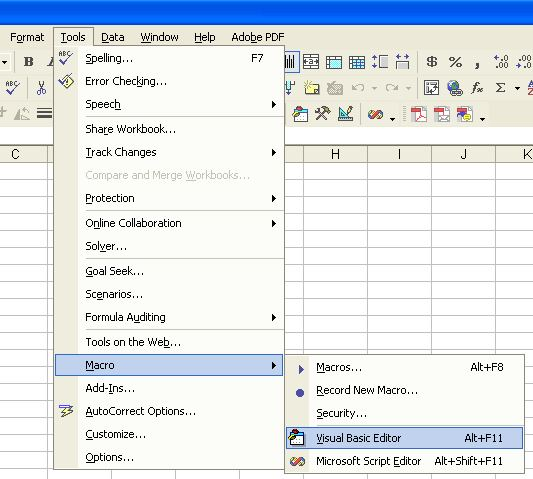2. In VBA Project, if you do not see any module, you may insert a new module by right click on the left pane and Insert-Module as shown in figure below. If you already have the module, ignore this step and go directly to the next step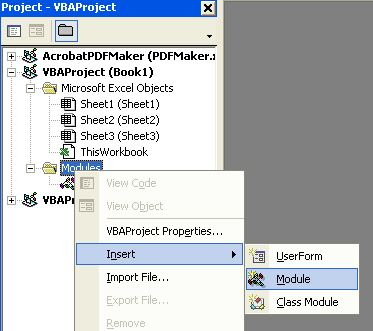3. Type your own function in the module. For example, you are going to make a function, which compute logical OR based on the binary digit of the number. VBA similar to visual basic can compute this function directly, but MS Excel will only return and false. Let us name this new function BinOr and it will take 2 inputs name a and b. The return value of this function is passed through calling the name of the function. See more explanation about BinOr function below.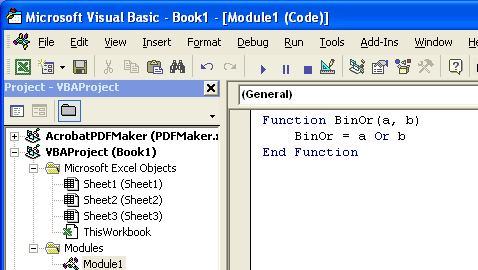4. Now you can close the Visual Basic Editor. How will you use your new function? You may type the name of the function directly inside your spreadsheet. For example, in cell B1 and B2 we input any number and cell B3 is the cell to write the formula, then the formula should be =BinOr(B1,B2)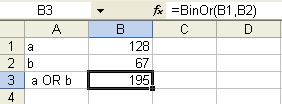5. Alternatively, you may use insert function toolbar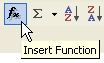or menu Insert-Function. If you press the Insert function toolbar, insert function dialog will show up. Click the category User Defined from the list and you will find the BinOr function is already in the list. Select the function and press OK button.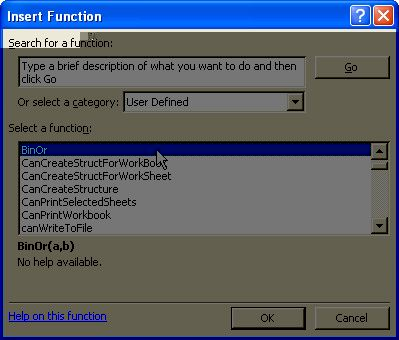6. Function argument dialog will show up and you may input the address of the two arguments or using mouse click. When you press OK button you get the result from your own user-defined function.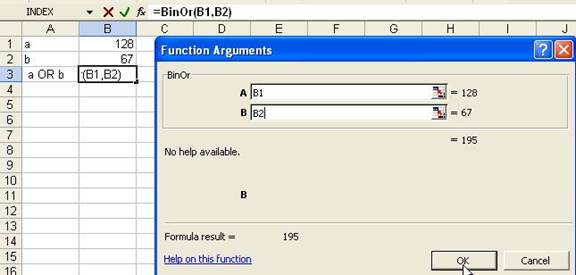Here is a little note about BinOr function. This function may be useful for image processing or cellular automaton simulation. If you use spreadsheet directly, OR function will return only either TRUE or FALSE. To get result as BinOr, you need to put your input as binary and then use logical OR for each binary digit and then put back the result to decimal.

Preferable reference for this tutorial is

Teknomo, Kardi (2006) How to write your own function in Microsoft Excel?. https:\\people.revoledu.com\kardi\ tutorial\Excel\UserDefinedFunction.html